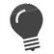# %BIT_IS_SET

Evaluate if a bit is set

 WTSupported in traditional Synergy on Windows WNSupported in Synergy .NET on Windows USupported on UNIX VSupported on OpenVMS
```status = %BIT_IS_SET(bit, value)
```

Return value

status

One of the following values: (n)

1 = The bit is set.

0 = The bit is clear.

Arguments

bit

The number of the bit to be evaluated. (n)

value

The value whose binary representation contains the bit to be evaluated. (n)

Discussion

%BIT_IS_SET evaluates a bit value in the binary representation of a value.

Bit numbering is zero-based. Bit can be 0 through 63 (or 31 on 32-bit systems), corresponding to values 1 through 8000000000000000 (hexadecimal).We recommend using bitwise Boolean operators rather than the %BIT_IS_CLR and %BIT_IS_SET functions.

Examples

The following example evaluates bit number 2 in the binary representation of 20:

```%bit_is_set(2,20)
```

Since the binary representation of 20 is 10100, and bit number 2 is actually the third bit from the right, this call to %BIT_IS_SET returns a value of 1.

The example below translates bit flags and displays their values to the terminal.

```subroutine show_bflags
a_bit_flags       ,n        ;Bit positions
.define PUBLIC_BIT       ,4
.define DOMESTIC_BIT     ,5
.define LESS50_BIT       ,6
.define VMAIL_BIT        ,7
proc
xcall showit("Public company?",
&       %bit_is_set(PUBLIC_BIT, a_bit_flags))
xcall showit("Domestic sales only?",
&       %bit_is_set(DOMESTIC_BIT, a_bit_flags))
xcall showit("Less than 50 employees?",
&       %bit_is_set(LESS50_BIT, a_bit_flags))
xcall showit("Voice mail system?",
&       %bit_is_set(VMAIL_BIT, a_bit_flags))
return
endsubroutine
subroutine showit
a_txt       ,a              ;Text to display
a_set       ,n              ;1 = set, 0 = not set
record
yn          ,a1             ;Y or N
proc
if (a_set) then
yn = 'Y'
else
yn = 'N'
display(TTCHN, a_txt, " (Y/N) ", yn)
return
endsubroutine```